On the Concentration Dependence of the Surface Tension of Liquid Metallic Alloys Theoretical Basis | OMICS International
Journal of Forensic Research

Like us on:

# On the Concentration Dependence of the Surface Tension of Liquid Metallic Alloys Theoretical Basis

Kaptay G1 and Papp Z2*

1Department of Physical Chemistry of the University of Miskolc, 3515, Miskolc, Egyetemvaros, Hungary

2University of Edinburgh, Division of informatics Scotland, UK

*Corresponding Author:
Zoltan Papp
University of Edinburgh, Division of informatics
Scotland, UK
Tel: +36 30 724 4609
E-mail: [email protected]

Received Date: July 20, 2015 Accepted Date: September 14, 2015 Published Date: September 21, 2015

Citation: Kaptay G, Papp Z (2015) On the Concentration Dependence of the Surface Tension of Liquid Metallic Alloys Theoretical Basis. J Forensic Res 6:310. doi: 10.4172/2157-7145.1000310

Copyright: © 2015 Kaptay G, et al. This is an open-access article distributed under the terms of the Creative Commons Attribution License, which permits unrestricted use, distribution, and reproduction in any medium, provided the original author and source are credited.

Visit for more related articles at Journal of Forensic Research

#### Abstract

In the present paper a revised version of Butler equation is derived to calculate the surface tension in multicomponent liquid metallic solutions. The effects of surface active complexes (intermetallic compounds) in liquid metallic solutions is also discussed.

#### Keywords

Liquid metallic alloys; Concentration dependence

#### Introduction

Surface tension is one of the basic thermodynamic properties of liquid metallic alloys, determining their behavior in different technological processes. Surface tension is determined by usual parameters of state – temperature and composition. Although there are very few experimental results on the concentration dependence of the surface tension of multi-component alloys, for complex modeling of different materials technologies this information is obviously needed. Therefore there is a need for a model, by which the surface tension of multi-component metallic alloys can be related to bulk thermodynamic properties. Such a model can serve as a basis for an ‘interfacial module’ in complex thermodynamic softwares.

In the present paper such a model will be derived from basic thermodynamic principles, and compared to the existing models in the literature. First, let us consider the models given in the literature so far, and then, let us derive our own equation.

#### Models Presented in the Literature

The surface tension of multi-component solutions is traditionally interpreted in terms of the Gibbs adsorption isotherms. For practical use, however, the method developed by Butler (based on Gibbs) is much more ‘user-friendly’ . According to Butler, the (partial) surface tension of component i in a multi-component solution can be written as: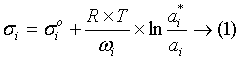Where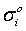is the surface tension of pure component i at the same temperature and pressure, R-gas constant,

T-absolute temperature,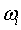-partial molar surface area of component i,

ai and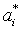-the activity of component i in the bulk and in the surface phase of the solution, respectively.

The surface phase will be in equilibrium, if all the components will have the same partial surface tension values, i.e. if the following equality is fulfilled between components i and j: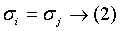For an n-component solution (n-1) equalities such as Eq (2) should be fulfilled. If the composition of the bulk solution is given, from those (n-1) equations the surface composition can be obtained, as it is characterized by (n-1) independent variables. For performing such a procedure, the following thermodynamic values are needed:

The surface tension of pure components as function of temperature,

The activities in the bulk phase, expressed through its composition and t,

The activities in the surface phase, expressed through its composition and t,

The partial molar surface area values expressed through the surface composition and t.

From the above list of initial parameters one can see that the new information needed for the evaluation of the concentration dependence of the surface tension isand. Instead of activity, usually the activity coefficient of component i in the surface is expressed, defined as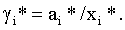. These values are usually expressed in the literature using the following approximations [2-10]: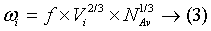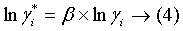where f-is the geometrical factor, usually used as 1.091 (taken equal to the value derived for the (111) plane of solid fcc crystals),

Vi - the partial molar volume of component i in the solution (for simplicity usually taken equal to the molar volume of pure component i),

β - a semi-empirical parameter, usually defined as the ratio of the broken bonds at the surface.

In different models parameter β is taken equal to different values for describing the surface tension of liquid metallic solutions. Hoar and Melford  used a value between β=0.5 and 0.667 to describe Sn-Pb and Pb-In systems. Monma and Sudo  applied a coefficient between β=0.80 and 0.84 for Cu-Ni and Ni-Mo systems. Speiser et al. [4,5] applied β=0.75 to describe Fe-Cu, Cu-Pb, Sn-Pb, Ag-Pb, Pb-In, Bi-Ag, Cu-Al, Fe-Si and Ni-Si systems. The same coefficients of β=0.75 was used by Lee et al.  for the Fe-Ni system. Tanaka et al. studied the influence of parameter β in the interval between 0.5 and 1 on the final results for Ag- Pb, Cu-Pb, Sn-Pb, Cu-Fe, Cu-Al, Ni-Si and various Fe-alloys . In the later paper of Tanaka et al.  the semi-empirical coefficient β=0.83 was suggested from the analysis of the dependency of the surface tension of pure liquid metals on their enthalpy of evaporation, and this coefficient was applied to the Cu-Pn and Fe-Si systems. The coefficient of β=0.83 suggested by Tanaka et al.  was successfully applied by Moser et al. to describe the Ag-Sn  and the Pb-Sn  systems.

#### The Model Developed in the Present Paper

In our model the Butler concept (Eqs 1 and 2) will be used as a basis, but it will be altered by considering separately the enthalpy and the entropy parts. Eq (3) also remains valid, but the value of coefficient f will be altered. The theory will be re-constructed from the very beginning, starting with the model for the surface tension of onecomponent liquid metals.

The surface tension of one-component liquid metals

By definition, the partial excess surface Gibbs energy of component i in the pure phase i can be obtained as the difference between the Gibbs energy of the surface and bulk phases: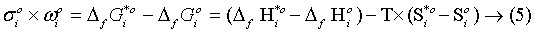The molar surface area of pure component i can be written in an analogous way with Eq (3):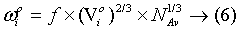The enthalpy and entropy differences between the surface and bulk phases in pure liquid metals can be written as: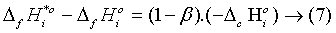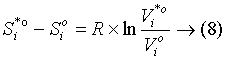where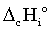-is the cohesion enthalpy of a pure liquid metal, being approximately equal to the condensation enthalpy (for a more advanced definition see ref. ),

β=z*/z, the ratio of the coordination numbers in the surface and bulk phases,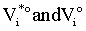are the molar volumes of pure component i in the surface and bulk phases, respectively.

According to our previous works [12,13], pure liquid metals have a surface structure close to the (111) plane of the fcc crystal, i.e. z*=9. Combining this value with the average coordination number in pure liquid metals (z ≅ 11), β ≅ 9/11=0.818. From the same surface model the value of parameter f in Eq. (6): f ≅ 1.06 [12,13]. The entropy difference in Eq. (8) approximately equals +4 J/molK [12,13]. The combination of these theoretically established parameters provide a perfect match with the experimentally determined surface tension values of pure liquid metals [12,13]. Let us mention, that these values are close to those applied by Tanaka et al.  (β=0.83, f=1.091, entropy contribution neglected).

Substituting Eqs (6-8) into Eq. (5) the final expression for the surface tension of pure component i can be written as: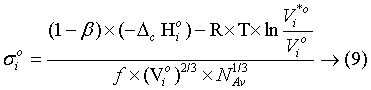The partial surface tension of component i in the multicomponent liquid metallic solution

For a partial surface tension of component i in the multi-component liquid solution Eq. (5) can be re-written, but with omitting the subscripto, referring to the pure phase: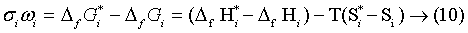The partial molar surface area of component i can be written by Eq. (3). However, we take f=1.06, being equal to the value of f derived for the pure liquid metal, and being independent of concentration. The partial molar volume is defined as: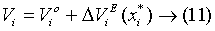where Δ ViE is the excess partial molar volume of component i, having the concentration dependence valid for the bulk phase, but being a function of the composition of the surface phase.

The enthalpy difference is composed of that in the pure phase, taking into account the partial mixing enthalpy terms (Δ Hi). The partial mixing enthalpy of the surface phase is a function of the surface composition, and is reduced by coefficient β, to take into account the reduced coordination number at the surface: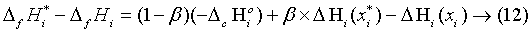The entropy term will include the term given above for the pure phase, the configurational entropy term and the partial excess mixing entropy term. None of these quantities should be multiplied by a correction factor of β (or any other coefficient), at least, in the first approximation: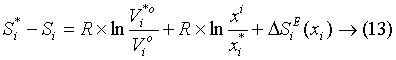Substituting Eq-s (11-13) into Eq. (10), and taking into account Eq. (9), the following final result is obtained: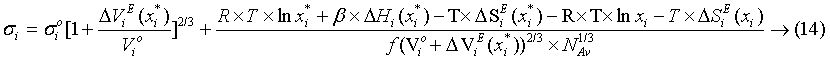Re-arranging Eq. (14) to be alike the Butler equation:

with the definition of γi*: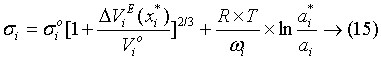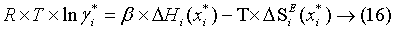One can see that Eqs (15 and 16) are different from Eqs (1 and 4). If Eqs (15 and 16) are combined with Eq. (2), the concentration dependence of surface tension can be calculated, supposing that the surface tension and molar volume of pure components are known as function of temperature, and all the excess functions (Δ Hi, Δ SiE, ΔViE) are known as function of composition of the bulk liquid metal and temperature. Generally, no analytical solution exists for this problem, and thus usually numerical methods are applied (see ref. ).

The analytical solution can be found only for the binary ideal solution A-B, if the two components have the same molar volume. Then, the composition of the surface phase and the surface tension can be calculated as: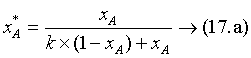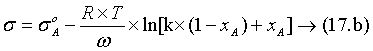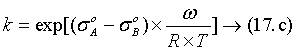The concentration dependence of the surface composition and the surface tension of a binary ideal solution are given in Figure 1. One can see that even in an ideal solution there is a great tendency to segregation of a component with a less surface tension value (component A) to the surface phase, leading to a very non-linear concentration dependence of the surface tension. As one can see from Figure 1, this tendency decreases with temperature, as at higher temperature the role of bulk configurational entropy is increased.

Figure 1: The concentration dependence of the surface composition (left) and surface tension (right) of the binary ideal solution (curves from bottom to top correspond to 500 K, 1,000 K and 1,500 K).

#### On the Role of Intermetallic Compounds on the Concentration Dependence of σ

As is obvious from phase diagrams, in many metallic phases intermetallic compounds can form. Some of those compounds melt congruently, and thus obviously remain stable even in a liquid state, especially, if there is a significant non-metallic bond exists between the components. Such systems are often described in the literature using the ‘associated solution model’ [15-17]. If there is a significant part of ionic bonding in the intermetallic compound, its own surface tension is usually below the surface tensions of its constituent pure metals . In these cases, minimum points on the concentration dependence of the surface tension are expected. However, no minimum points can be described by the formalism given above.

In order to describe mathematically the systems with associates, an associate solution model should first be applied. Then, in the binary system, at least three quasi-components (the two monomers and at least one associate) will exist. The concentration dependence of such a system can be described only, if the surface tension of the pure liquid intermetallic is known, and the system is treated as a 3-component system. For this first the equilibrium bulk concentrations of the three components should be found from bulk thermodynamic values [15-17]. If the surface tension of the liquid intermetallic is not known, it can be estimated by a fitting procedure, using experimental data.

#### Conclusions

The Butler equation has been revised in the present paper to describe more correctly the concentration dependence in multicomponent liquid metals. Calculations are performed in ref. . The hypothesis is given about the possible role of intermetallic compounds on the minimum points observed on the concentration-surface tension curves. Numerical calculations will be performed in the future to prove this hypothesis.

#### References

Select your language of interest to view the total content in your interested language

### Article Usage

• Total views: 12674
• [From(publication date):
September-2015 - Jan 19, 2020]
• Breakdown by view type
• HTML page views : 8821Can't read the image? click here to refresh